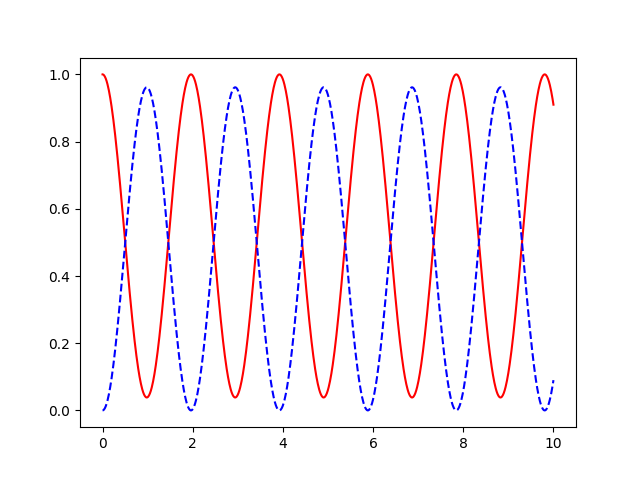# The Analytical Solution of Rabi Cycling in the Two-Level-System¶

In :
import matplotlib.pylab as plt
import numpy as np
from numpy import pi as π
from numpy import exp, cos, sin, sqrt, abs

In :
%matplotlib notebook

In :
from IPython.display import display

In :
%load_ext version_information
%version_information numpy, matplotlib, sympy

Out:
SoftwareVersion
Python3.7.3 64bit [GCC 7.3.0]
IPython7.8.0
OSLinux 4.14.138+ x86_64 with debian buster sid
numpy1.18.1
matplotlib3.1.2
sympy1.5.1
Tue Jan 28 23:34:30 2020 UTC

## Check the analytical solution to the TLS¶

We consider the solution of the Schrödinger equation for the two level system in the rotating wave approximation \begin{equation} i \hbar \frac{\partial}{\partial t} \begin{pmatrix} b(t) \\\\ a(t) \end{pmatrix} = \begin{pmatrix} 0 & \frac{\Omega_0}{2} \\\\ \frac{\Omega_0}{2} & \Delta\end{pmatrix} \begin{pmatrix} b(t) \\\\ a(t) \end{pmatrix} \end{equation} with the boundary condition $a(0) = 1$, $b(0) = 0$. Here and everwhere else, we work in energy units of $2 \pi$ GHz and time units of ns, and thus have $\hbar = 1$

In :
import sympy as sp
from sympy import I, diff
sp.init_printing()


We "guess" the analytical solutions (based on D. Tannor, "Introduction to Quantum Mechanics: A Time-dependent Perspective", with some small corrections):

In :
Δ, Ω0, t, Ω = sp.symbols((r'\Delta', r'\Omega_0', 't', r'\Omega'), real=True)
#Ω = sp.sqrt(Δ**2 + Ω0**2)
a = sp.exp(-I*Δ*t/2)*( sp.cos(Ω*t/2) - I*(Δ/Ω)*sp.sin(Ω*t/2) )
b = -I * (Ω0/Ω)*sp.exp(-I*Δ*t/2)*sp.sin(Ω*t/2)

In :
a

Out:
$\displaystyle \left(- \frac{i \Delta \sin{\left(\frac{\Omega t}{2} \right)}}{\Omega} + \cos{\left(\frac{\Omega t}{2} \right)}\right) e^{- \frac{i \Delta t}{2}}$
In :
b

Out:
$\displaystyle - \frac{i \Omega_0 e^{- \frac{i \Delta t}{2}} \sin{\left(\frac{\Omega t}{2} \right)}}{\Omega}$

and check that the left hand side of the Schrödinger Equation equals the right side

In :
sp.simplify((diff(a, t) + I*(Ω0/2)*b + I*Δ*a).subs({Ω:sp.sqrt(Δ**2 + Ω0**2)}))

Out:
$\displaystyle 0$
In :
sp.simplify(diff(b, t) + I*(Ω0/2)*a)

Out:
$\displaystyle 0$

## Ideal π-pulse¶

Population transfer is achieved for $\Omega t = \pi$. The amplitudes in the ideal case of no detuning are:

In :
a.subs({Ω * t: sp.pi, Δ:0})

Out:
$\displaystyle 0$
In :
b.subs({Ω * t: sp.pi, Δ:0, Ω0: Ω})

Out:
$\displaystyle - i$

Note the non-zero phase on $b$: Even a $2 \pi$ pulse (a full cycle) will not reproduce the initial state, but result in a $\pi$-phase shift. Only two full cycles reproduce the exact original state.

For $\Delta \neq 0$, the phases behave in a considerably more complicated way. In particular, the imaginary part of $a$ (i.e., the phase of $\vert0\rangle$) will no longer be zero:

In :
a.subs({Ω * t: sp.pi})

Out:
$\displaystyle - \frac{i \Delta e^{- \frac{i \Delta t}{2}}}{\Omega}$
In :
b.subs({Ω * t: sp.pi})

Out:
$\displaystyle - \frac{i \Omega_0 e^{- \frac{i \Delta t}{2}}}{\Omega}$

## Compare analytical and numerical solution of TLS¶

We now simulate the dynamics of the two-level system numerically, and compare the result to the analytical solution

In :
Ω0 = 2*π*0.5 # 2π GHz
Δ = 2*π*0.1 # 2π GHz

In :
def a(Ω0, Δ, t):
"""Complex amplitude of the TLS solution, for the \ket{1} component"""
Ω = sqrt(Δ**2 + Ω0**2)
return exp(-0.5j*Δ*t)*( cos(0.5*Ω*t) - 1j*(Δ/Ω)*sin(0.5*Ω*t) )

In :
def b(Ω0, Δ, t):
"""Complex amplitude of the TLS solution, for the \ket{0} component"""
Ω = sqrt(Δ**2 + Ω0**2)
return -1j*(Ω0/Ω)*exp(-0.5j*Δ*t)*sin(0.5*Ω*t)

In :
def show_rabi_cycling(Ω0, Δ, tgrid):
fig = plt.figure()
a_s = a(Ω0, Δ, tgrid)
b_s = b(Ω0, Δ, tgrid)
ax.plot(tgrid, abs(a_s)**2, label='pop(1)', color='red')
ax.plot(tgrid, abs(b_s)**2, label='pop(0)', color='blue', ls='--')
fig.show()

In :
show_rabi_cycling(Ω0, Δ, np.linspace(0, 10, 1000))In :
H = np.matrix([[0, 0.5*Ω0],[0.5*Ω0, Δ]], dtype=np.complex128); H

Out:
matrix([[0.        +0.j, 1.57079633+0.j],
[1.57079633+0.j, 0.62831853+0.j]])
In :
evals, W = np.linalg.eig(H)

In :
def get_U(evals, W, dt):
"""Get the time evolution operator for a single time step"""
D = np.matrix(np.diag(np.exp(-1j*evals*dt)))
W = np.matrix(W)
return np.asarray(W*D*W.H)

In :
def propagate(psi, tgrid, evals, W):
"""Propagate psi along the given time grid, assuming a constant
Hamiltonian with the given eigenvalues and eigenvector matrix.

Returns an array of shape (2,nt) that gives the propagated
amplitude at each point in time."""
nt = len(tgrid)
result = np.zeros((2, nt), dtype=np.complex128)
tgrid = list(tgrid)
dt = tgrid - tgrid
i = 0
U = get_U(evals, W, dt)
while len(tgrid) > 0:
tgrid.pop()
result[:, i] = psi
psi = U.dot(psi)
i += 1
return result

In :
dyn = propagate(np.array([0,1]), tgrid=np.linspace(0,10,1000), evals=evals, W=W)

In :
def show_dyn(dyn, tgrid):
fig = plt.figure()
ax.plot(tgrid, abs(dyn[1,:])**2, label='pop(1)', color='red')
ax.plot(tgrid, abs(dyn[0,:])**2, label='pop(0)', color='blue', ls='--')
fig.show()

In :
show_dyn(dyn, np.linspace(0, 10, 1000))The maximum error between the analytical and numerical solution is:

In :
print(np.max(np.abs(dyn[0,:] - b(Ω0, Δ, np.linspace(0, 10, 1000)))))

2.29480091309186e-13

In :
print(np.max(np.abs(dyn[1,:] - a(Ω0, Δ, np.linspace(0, 10, 1000)))))

2.7207552755516735e-13